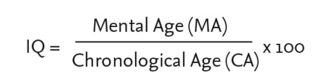# intelligence quotient

(redirected from WISC-III test)
Also found in: Dictionary, Thesaurus, Medical.

## intelligence quotient

a measure of the intelligence of an individual derived from results obtained from specially designed tests. The quotient is traditionally derived by dividing an individual's mental age by his chronological age and multiplying the result by 100

## intelligence quotient (IQ)

a unit used in the field of INTELLIGENCE measurement and testing as an index of an individual's intelligence relative to a comparable population with respect to age. A ratio IQ is the IQ expressed as a ratio of mental age (as measured by a test) to chronological age, and multiplied by 100 to avoid decimals:The average child at any one chronological age will therefore score 100 on the appropriate set of IQ test items. This was the original IQ measure first used in 1916 in the Stanford-Binet Test.

Modern tests make use of standard scores, which express the individual's distance from the mean in terms of the standard deviation, and assume a normal distribution. In a variant of this, the deviation IQ, the mean is 100 and a standard deviation of 15 or 16 is usual.

It is important to note the difference between these measures, since the deviation IQ is not a ratio of mental age to chronological age, and the measured IQs derived from it will depend on the standard deviation used in the test. see also INTELLIGENCE TEST.

## intelligence quotient

[in′tel·ə·jəns ‚kwōsh·ənt]
(psychology)
The numerical designation for intelligence expressed as a ratio of an individual's performance on a standardized test to the average performance according to age. Abbreviated IQ.
Site: Follow: Share:
Open / Close